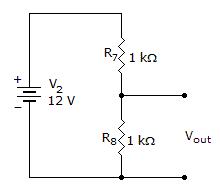# Electronics - Series-Parallel Circuits

### Exercise :: Series-Parallel Circuits - True or False

21.

The voltage out when a 10 kload is connected is 5.7 volts.A. True B. False

Explanation:

No answer description available for this question. Let us discuss.

22.

When resistors burn out, they usually short.

 A. True B. False

Explanation:

No answer description available for this question. Let us discuss.

23.

Attaching a 6 kload to a voltage divider will cause a smaller decrease in the output voltage than will attaching a 5 kload.

 A. True B. False

Explanation:

No answer description available for this question. Let us discuss.

24.

A loaded voltage divider is a common application of a series-parallel circuit.

 A. True B. False